IT

# Understanding and Baselining Network Behaviour using Machine Learning - Part II

A difficult question we come across with many customers is ‘what does normal look like for my network?’. There are many reasons why monitoring for changes in network behaviour is important, with some great examples in this article - such as flagging potential security risks or predicting potential outages.

It can often be hard to define baseline behaviour or performance KPIs for a given network metric - especially if your organisation has just seen major changes to a service such as a shift to working from home. Here we will present a few methods for developing baseline thresholds.

## Baselining Network Behaviour

Hopefully, you have had a chance to read the first part of this blog series that focussed on understanding your network. In this second installment we will continue to use the Coburg Intrusion Detection Data Sets (CIDDS) to determine baseline behaviour for one of the nodes we identified as critical in the first half of this series.

Let’s take the IP address 192.168.220.15 that we identified as a critical node on our network using graph analytics. The issue we have is that we don’t know what normal looks like for this source IP.

Starting with a time chart we can view the total number of flows to and from this IP address with the following search:

```| tstats sum(Flows) as sum_flows WHERE (index=cidds "Src IP Addr"=192.168.220.15) BY _time span=5m
```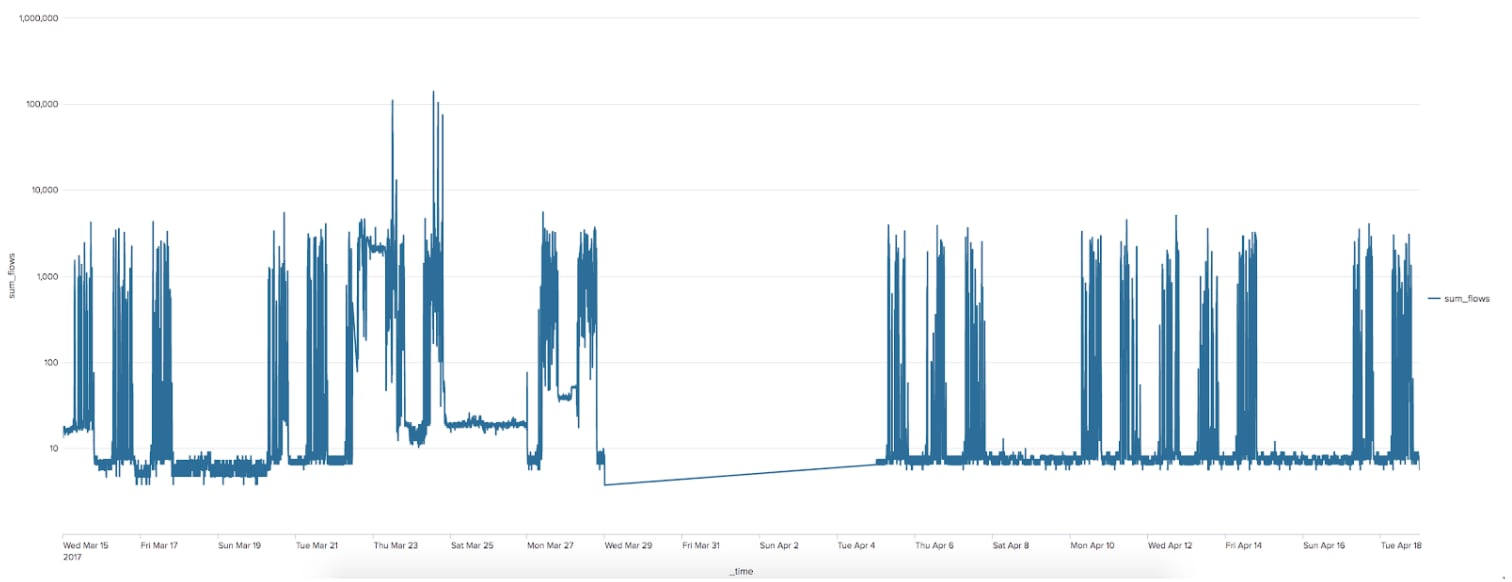It is important to note that this chart has been plotted against a log scale - there is a high degree of variance in the number of flows, and the baseline behaviour is much easier to see on this scale. Please see this great link to brush up on your knowledge of logarithms.  We can see from the visual that there is a pattern of activity during weekdays and weekends, with increases during the daytime for a weekday and limited activity over weekends. There is also a period of complete inactivity, which suggests a maintenance window for this server or network device. In reality this is a gap in the CIDDS data caused by a power cut at Coburg University over a few days when they were generating the data!

We’re now going to look at three techniques for generating a baseline for this behaviour, which increases in complexity as we progress through the blog.

### Baseline 1: Using Statistics

In order to generate a baseline for this data we are going to apply a couple of approaches, the first of which is described in more detail in the blog here. Essentially we are going to use the average number of flows and standard deviation of those to determine a few boundary ranges based on the time chart, where any points that sit outside the boundary range will be flagged as outliers. The search for this can be seen below:

```| tstats sum(Flows) as sum_flows WHERE (index=cidds "Src IP Addr"=192.168.220.15) BY _time span=5m
| eval HourOfDay=strftime(_time, "%H"), DayOfWeek=strftime(_time, "%A")
| eval Weekday=if(DayOfWeek="Saturday" OR DayOfWeek="Sunday","No","Yes")
| eventstats avg("sum_flows") as avg_f stdev("sum_flows") as stdev  by "HourOfDay", "Weekday"
| eval lower_bound=(avg_f-stdev*exact(3)), upper_bound=(avg_f+stdev*exact(3))
| eval isOutlier=if('avg' < lowerBound OR 'avg' > upperBound, 1, 0)
| table _time sum_flows lower_bound upper_bound
```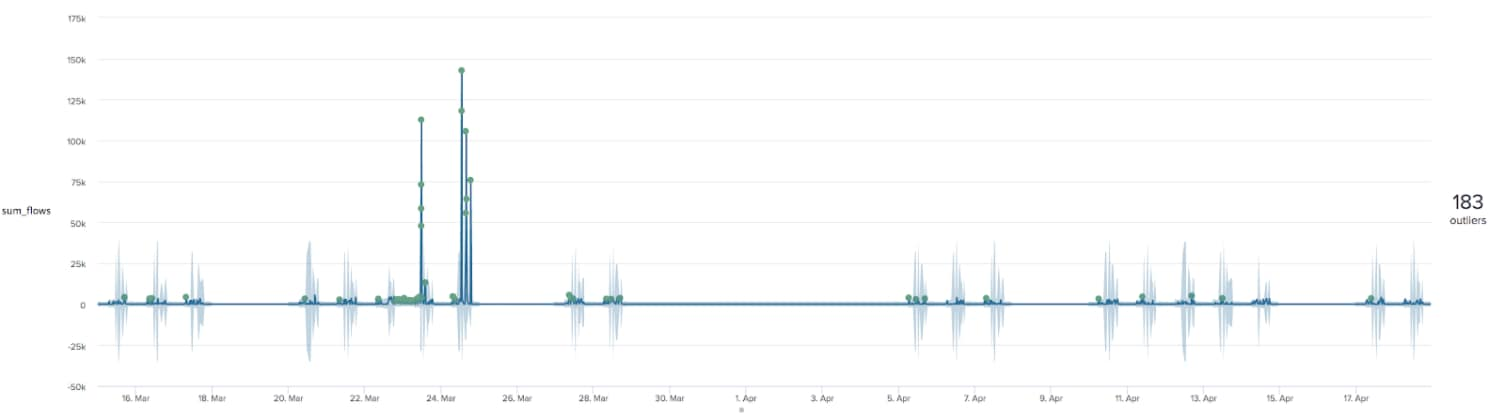As can be seen from the results, this search has generated a light blue range that represents the expected values at a given time of day for the average duration of connections to this IP address. In other words, we have a baseline for what is ‘normal’ for this data. If we were to persist this baseline as a lookup (as described in the cyclical statistical forecasts and anomalies blog linked above) we could then compare new data to the baseline and flag any potential performance issues as they are happening. This provides a glimpse into a potential issue in the near future, allowing you to act now to minimise or even stop an outage from occurring in the first place.

### Baseline 2: Using Probability Density

Another method for determining a baseline for your data is using the Density Function. This is similar to the search using averages and standard deviations above, but can model more complex data. The search below demonstrates how to apply this technique:

```| tstats sum(Flows) as sum_flows WHERE (index=cidds "Src IP Addr"=192.168.220.15) BY _time span=5m
| eval HourOfDay=strftime(_time, "%H"), DayOfWeek=strftime(_time, "%A")
| eval Weekday=if(DayOfWeek="Saturday" OR DayOfWeek="Sunday","No","Yes")
| fit DensityFunction sum_flows by "Weekday,HourOfDay" as outlier into df_192_168_220_15 threshold=0.003
```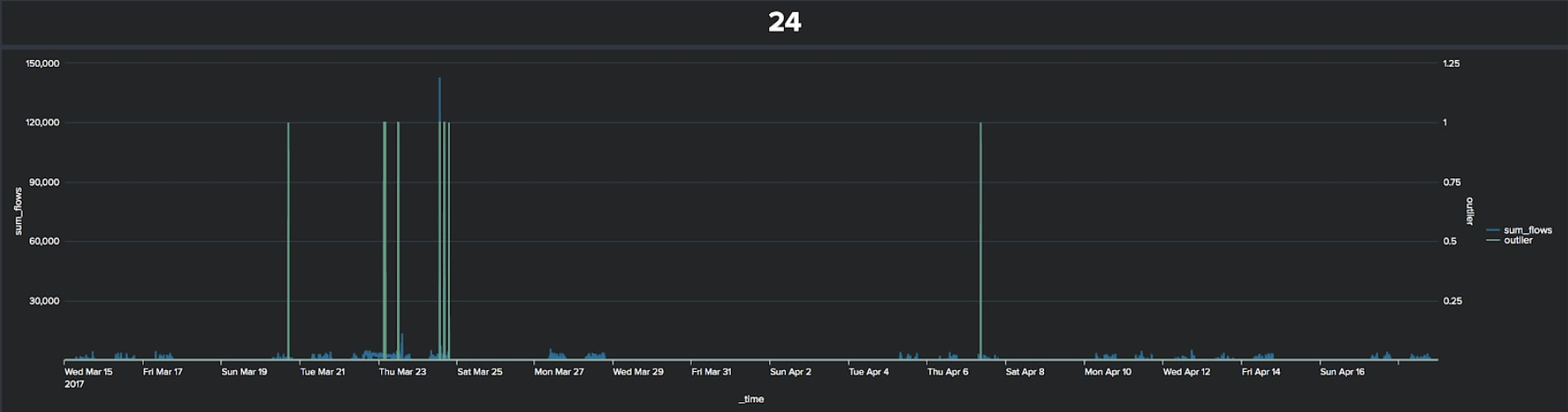In the results here - with a threshold of 0.003, which is equivalent to three standard deviations if the data is normally distributed - we have significantly less anomalies than from the previous technique. Note that the majority of the anomalies occur in the second week of the chart, as for the first approach.

### Baseline 3: Using Regression

Let’s look at one final method for determining baseline behaviour for a network using the System Identification algorithm, which is a regression algorithm based on a densely connected neural network. When applying this algorithm we are going to increase the complexity of our model quite a bit by including several other metrics from our NetFlow data, namely the average number of bytes transferred, the total bytes transferred, the average duration of the connections, the number of packets transferred, and the number of distinct connections.

The search to apply the System Identification algorithm is below, which produces three additional fields:

• A predicted value for the number of flows
• An upper bound for the number of flows
• A lower bound for the number of flows
```| tstats sum(Flows) as sum_flows avg(Bytes) as avg_bytes sum(Bytes) as sum_bytes avg(Duration) as avg_duration sum(Packets) as sum_packets dc("Dst IP Addr") as distinct_connections WHERE (index=cidds "Src IP Addr"=192.168.220.15) BY _time span=5m
| eval HourOfDay=strftime(_time, "%H"), DayOfWeek=strftime(_time, "%A")
| eval Weekday=if(DayOfWeek="Saturday" OR DayOfWeek="Sunday",0,1)
| fit SystemIdentification sum_flows from avg_bytes sum_bytes avg_duration sum_packets distinct_connections HourOfDay Weekday dynamics=3-3-3-3-3-3-3-3 conf_interval=99 into si_dur_cidds
```

Note the dynamics variable in the fit command, which is telling us to include the latest 3 values of each variable when training and applying the model. In other words, this algorithm is calculating the likely value for the current number of flows based on the past 15 minutes of data, rather than a single 5 minute window calculated in the tstats command. This can be a really useful technique when modelling data that has a delay between one variable and another.  For example, when boiling water in a pan there is usually a delay between turning up the temperature of the hob and all of the water heating up.

Because this technique is for predicting numeric value (an example of supervised learning) we have split the data into two sections:

• A training set
• A testing set

In the visual below you can see the results of the fit command on the training set, which is the later two weeks of data in the CIDDS data set - i.e. the data from the 5th April onward.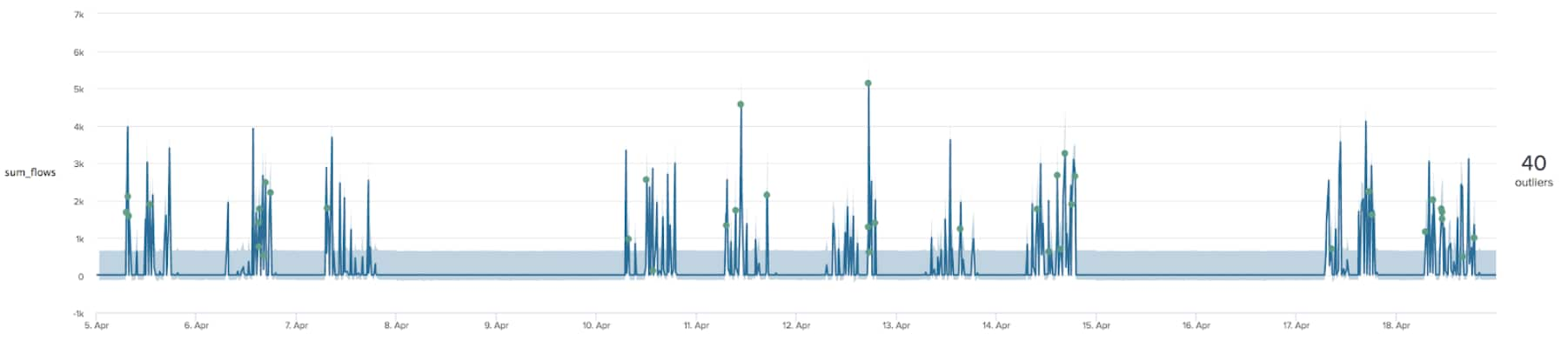When applying our trained model to the first two weeks of data we produce the chart below - where there are significantly more outliers (as seen when applying both of the previous two techniques).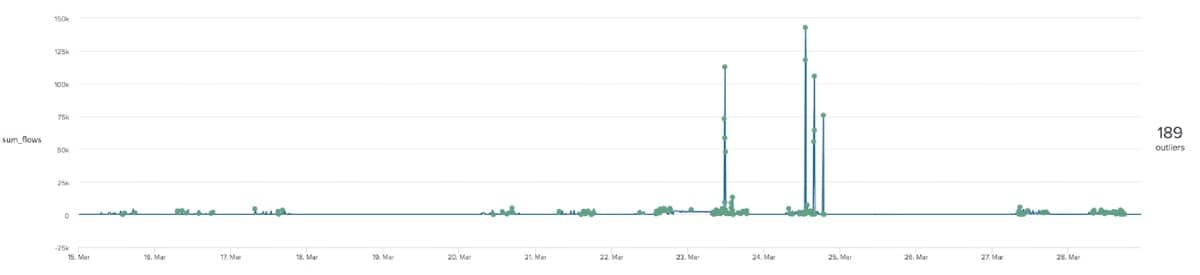Although we are generating many more alerts than our previous methods we are also modelling much more complex data with this model. For this example we may have produced an overly complex model, where the simpler techniques outlined above are more appropriate in this instance.

It is not just the count of outliers that is of interest here. We also need the accuracy metrics from our second stage where we apply the data. We can see that we are getting an R2 or R-squared score of around 0.7, this suggests our predictive model is 70% accurate. R-squared is a statistical measure of how close the data are to the fitted regression line.We are also getting a root mean squared error of around 2.5k, which tells us that our predictions are usually within 2.5k out from the actual values. Since our data goes to almost 150k in some places this isn’t too bad!## Summary

We have shown a few supervised and unsupervised methods for baselining network behaviour here. All three techniques we have applied highlight a large number of outliers in the second week of the dataset, though differ in the number of outliers that are identified. With thanks again to Markus and Sarah of Coburg University, what we have found here in the second week of the data reflect the fact that the IP 192.168.220.15 was used as the internal attack server for that week, highlighting that we have identified a security risk using these techniques.

In fact, returning to some of the graph analytics techniques used in the previous blog post we can actually see how the behaviour of the IPs 192.168.220.15 and 192.168.220.16 change over time as they are used as the attack servers. In the visual below you can see how the profile of the network changes week-by-week using graph analytics - with both of the highlighted IP addresses having high degrees of connectivity during the weeks they are used as attackers. Therefore taking baseline snapshots of the centrality measures for each IP on the network could be another way of monitoring baseline behaviour of a network.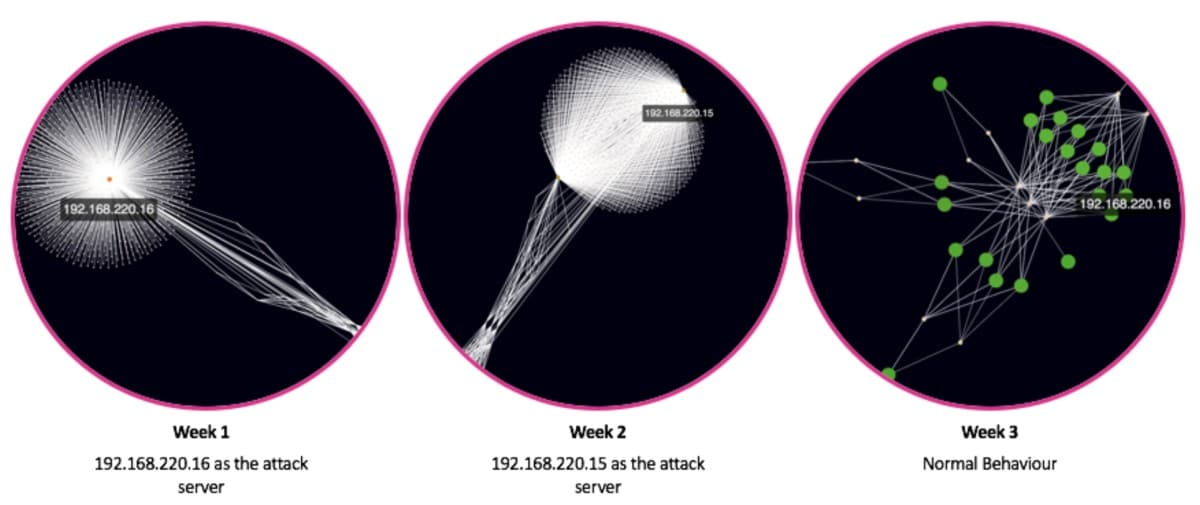If you want to take the next step in analysis and actually start predicting the future values for a given metric please read this blog, which describes the predictive modelling technique that we use in ITSI

Hopefully you will be able to take some of these techniques and apply them to your network data to get a better understanding of how it is performing, especially in these times of dramatic change.

Happy Splunking.

Special thanks to Philipp Drieger and Bryan Sadowski for their help collating the material for this blog series, and also to Markus Ring and Sarah Wunderlich from Coburg University for their valuable insight into the CIDDS data and their ongoing research.Posted by

#### Greg Ainslie-Malik

Greg is a Machine Learning Architect at Splunk where he helps customers deliver advanced analytics and uncover new ways of insight from their data. Prior to working at Splunk he spent a number of years with Deloitte and before that BAE Systems Detica working as a data scientist. Before getting a proper job he spent way too long at university collecting degrees in maths including a PhD on “Mathematical Analysis of PWM Processes”. When he is not at work he is usually herding his three young lads around while thinking that work is significantly more relaxing than being at home…

TAGS

Show All Tags
Show Less Tags